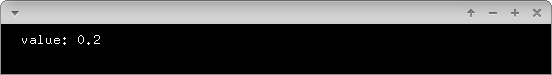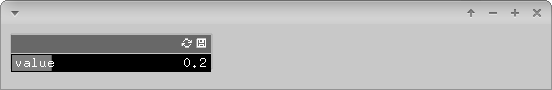# View the value of a variable

There are multiple ways on how to display values of a variable.

## Console output

The following examples will show you how you can create output at the console.

### Using std::cout

Probably the simplest way is to use std::cout. This command lets you combine different types of values with strings. Appending `endl` creates a line break.

``````float value = 0.2;
std::cout << "value: " << value << endl;
``````

Output:

``````value: 0.2
``````

### Using std::printf

printf can be used to force all sorts of different output formats. `%f` is a placeholder for a float variable you are appending. `%.0f` and `%.3f` set the decimal places of the printed value. `\n` creates a line break. Have a look at the reference for details and examples.

``````float value = 0.2;
std::printf("value: %f\n", value);
std::printf("value: %.0f\n", value);
std::printf("value: %.3f\n", value);
``````

Output:

``````value: 0.200000
value: 0
value: 0.200
``````

### Using ofLog()

The best way to integrate with the openFrameworks workflow is to use the implemented logging functions. There are different log levels and multple ways of usage - have a look at the ofLog() documentation. Here is one example:

``````float value = 0.2;
ofLogNotice() << "value: " << value;
``````

Output:

``````[notice ] value: 0.2
``````

You can also redirect the log messages to a file:

``````ofLogToFile("myLogFile.txt", true);
// all following logs will be appended to myLogFile.txt
``````

## Graphical output

### Drawing Text

Drawing text to the screen is as simple as this:

``````void draw() {
ofBackground(ofColor::black);
}

void draw() {
float value = 0.2;
ofSetColor(ofColor::white);
ofDrawBitmapString("value: " + ofToString(value), 10, 10);
}
``````### Using ofxGui

Another nice way of viewing your variable that also gives you the ability to change it is using ofParameter and the core addon ofxGui. Read how to add an existing addon for details on how to add ofxGui to your project.

In the header file, wrap your variable with ofParameter. You can still work with this variable as you were used to, but it makes you able to add listeners to the variable or to add the variable to a GUI that will interact with it.

``````//ofApp.h

#include "ofxGui.h"
#include "ofMain.h"

class ofApp : public ofBaseApp {

..

ofParameter<float> value;
ofxPanel gui;

}
``````

In the source file, you can give the value a name, a default value and minimal / maximal borders (in case of numerical values). You have to setup the GUI and add the value, draw the GUI. You will then be able to interact with the value.

``````//ofApp.cpp

void setup() {
value.set("value", 0.2, 0, 1); // name, default value, min, max
gui.setup();